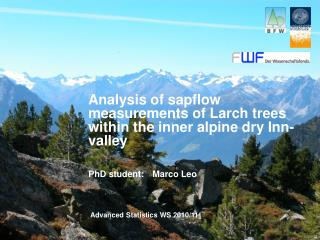DownloadDownload PresentationAnalysis of sapflow measurements of Larch trees within the inner alpine dry Inn-valley

# Analysis of sapflow measurements of Larch trees within the inner alpine dry Inn-valley

Télécharger la présentation## Analysis of sapflow measurements of Larch trees within the inner alpine dry Inn-valley

- - - - - - - - - - - - - - - - - - - - - - - - - - - E N D - - - - - - - - - - - - - - - - - - - - - - - - - - -
##### Presentation Transcript

1. Analysis of sapflow measurements of Larch trees within the inner alpine dry Inn-valley PhD student: Marco Leo Advanced Statistics WS 2010/11

2. Overview • Background • Principleofsapflowmeasurements • Collectionof environmental data • Statistical analysisof time seriesdata • Descriptivestatistics • Multiple linear regression • Autocorrelation

3. Principle of sapflow measurements • Two sensors installed into the sapwood • The top sensor is heated • Temperature difference between the sensors • Calculation of the sapflow density [ml cm2 min] • Relative sapflow for data interpretation ! • Dependent variable

4. Dependence of environmental parameters • Collected environmental data: (independent variables)

5. Typical sesonal course of sapflow density

6. Box plots I

7. Box plots II

8. Scatter plots

9. Multiple linear regression (model VPD2)

10. y vs. fitted and residuals vs. time

11. What is Autocorrelation ? Autocorrelation is the correlation of a signal with itself (Parr 1999). part of the data:

12. Testing Autocorrelation Durbin Watson Test H0 : α = 0 → No Autocorrelation H1 : α ≠ 0 → Autocorrelation durbinWatsonTest(model_LA_2) lag Autocorrelation D-W Statistic p-value 1 0.5097381 0.9703643 0 Alternative hypothesis: rho != 0

13. Determine the strength of the Autocorrelation • Autocorrelation Function (ACF) • Partial Autocorrelation Function (PACF) Yt = α Yt-1 + εt

14. Time series model - ARIMA • Elimination of the Autocorrelation • Results: • Summary • Table with coefficients and standard errors

15. Residual plots

16. ACF and Partial ACF

17. Multicollinearity • Variance Inflation Factors (vif) • tolerance = 1/vif

18. Differential effect of the independent variables bj…regression coefficient Sxj…standard deviation of xj Sy…standard deviation of y

19. Optimal VPD for sapflow

20. Helpful R commands/featuresforusing time seriesdata: • Arima model: the output differs from a lm model • Residual diagnostic • plot(model_LA_2\$resid,xlab="day of year",main="VPD2 model“) • Create lines to get an overview of diagnostic plots • abline(h=0,col="red") • abline(0,1,col="red")

21. Thank you for your attention !